# Electronics and Communication Engineering - Networks Analysis and Synthesis

1.

Substitution theorem applies to

 A. Linear network B. Non linear network C. Linear time invariant network D. Any network

Explanation:

No answer description available for this question. Let us discuss.

2.

In figure, i = Im sin ωt. If v = 0, ω =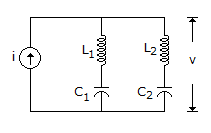A.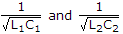B.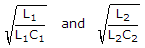C.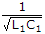D.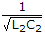Explanation:

No answer description available for this question. Let us discuss.

3.

An R-L circuit has an initial current of 5 A. In the s-domain equivalent circuit, the voltage source to take into account the initial current has a strength of

 A. 5L volts B. 5/L volts C. 5L2 volts D. 5/L2 volts

Explanation:

No answer description available for this question. Let us discuss.

4.

A function f(t) = k is

 A. stable B. marginal stable C. unstable D. none

Explanation:

No answer description available for this question. Let us discuss.

5.

Transient current in a loss free LC circuit when excited from an ac source is an __________ sine wave.

 A. undamped B. over damped C. under damped D. critically damped